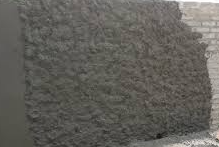### What is brass in civil construction? Its conversion and weight calculation.

Brass is a non-standard unit that is still prevalent in India with a history of 200 years or so. The word brass is used as a measurement unit, that represents the multiple of 100 for both the volume and the area in the British system.

Now let us go through, some of the FAQs related to the term brass in the civil field.

What is brass in civil construction?

The term brass means 100 cubic feet when we calculate the volume of any building materials in the construction field, and it is also equivalent to 100 square feet when we estimate the surface area.

When the building materials like sand, metals, moorum, boulders, etc. are brought on the construction site by trucks, dumpers, tractors, or any such vehicles,  we denote the volume by the word brass.In context, the area covered by the flooring, tiles, plaster, paintings, etc. in the buildings, is also represented by the same word, i.e. brass.

How to convert one cubic meter into brass?

As you know,  1brass = 100 cubic ft.

and 1cubic meter= 35.315 cubic feet.

So, 1 brass = 100/35.315 = 2.831 cubic meter.

Inversely, 1 cubic meter = 35.315/100 =0.353 brass.

What is meant by one brass material in a truck?

Suppose if the size of the truck container is  14ft.in length (L), 7ft.in width (W), and 3ft. in depth(D), then the volume of the container = L*W*D = 14ft*7ft*3ft = 294 cubic ft.

Now, 1 brass = 100 cubic ft. So, converting the cubic ft. into brass i.e. 294/100 = 2.94 brass.

When the truck of the above container size supplies the sand up to the brim, then we measure it as 2.94 brass of sand.

How to calculate the weight of one brass of sand?

The weight of one cubic meter of sand is 1630kg. (approx.)

1 brass = 2.831cubic meter (we calculated above).

So, the weight of 1 brass of sand = 1630*2.831= 4615kg. i.e. 4.615ton.

Similarly, we can calculate the weight per brass of other building materials.

To know why the desert & sea sand is not used in construction, go through the article👇

What is meant by one brass of plaster?

Suppose if the surface of the wall plastered is 100 sq. ft. in area, then we call it one brass of plaster.For eg., if the plastered area of the wall is 25ft in length and 10ft. in height then the area we get is 25ft.×10ft.= 250sq.ft.

To convert it into brass we have to divide the value by 100, i.e. 250 ÷100 =2.5 brass. of plaster.

Suppose if the above-given measurement is in meters, then the area we get is

25m × 10m = 250sqm.= (250× 10.764 ) sqft = 2691sqft.

To convert it into brass we have to divide the value by 100, i.e. 2691 ÷  100 =26.91 brass. of plaster.

or  250sqm ÷ 9.29 =26.91brass ( 1sqm = 9.29 brass )

In the same way, you can convert the area of the flooring, painting, punning, etc. into brass and vice versa.

#### Brass conversion for the areas.

100sqft. = 1brass.

100sqm = 10.764brass

Inversely, 1brass = 9.29 sqm.

If you have any questions regarding brass and its calculation procedure, you can ask me in the comment section.

Subscribe(free) or visit again

Have a good day.😄

1.we have to construct fencing wall of size Meter 158.58 L* m 2.12H*m *m 0.60
can u please tell how much brass ?

2.158.58m × 2.12m = 336.189sqm.÷ 9.29 = 36.189 brass in area
158.58m × 2.12m × 0.6m = 201.71 cum.÷ 2.831 = 71.25brass by volume.

3.Civil Engineering Spreadsheets Thank you because you have been willing to share information with us. we will always appreciate all you have done here because I know you are very concerned with our.

4.Thank u for giving useful information

5.whirlpool 7.2 kg semi automatic top load washing machine price

6.